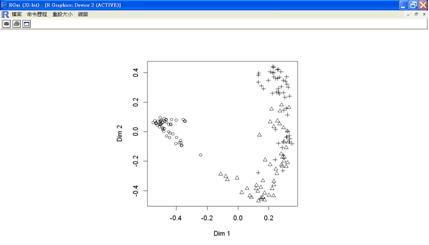﻿ R統計分析與資料探勘入門—以鳶尾花資料集為例

# ntuccepaper2019

## 專題報導

### 手機版選單

#### 字級

R統計分析與資料探勘入門—以鳶尾花資料集為例
• 卷期：v0031
• 出版日期：2014-12-20

R軟體提供了眾多的套件，往往讓初學者無所適從，因此在這裡我們將以一個R內建的鳶尾花資料集為例，說明整個分析處理過程，包括敘述統計、繪圖功能、常態性檢定、雙樣本均數檢定、變異數分析、迴歸分析、決策樹資料探勘等分析應用，讓初學R軟體的人可以很快地掌握整個統計分析的處理過程。

### 前言

R軟體提供了數量龐大的套件，往往讓初學者無所適從，因此在這裡我們將以一個R內建的鳶尾花資料集為例，說明整個分析處理過程，包括敘述統計、繪圖功能、常態性檢定、雙樣本均數檢定、變異數分析、迴歸分析、決策樹資料探勘等分析應用，讓初學R軟體的人可以很快地掌握整個統計分析的處理過程。

### 一. 敘述統計

1. 花萼長度(Sepal Length)：計算單位是公分。
2. 花萼寬度(Sepal Width)：計算單位是公分。
3. 花瓣長度(Petal Length) ：計算單位是公分。
4. 花瓣寬度(Petal Width)：計算單位是公分。
5. 類別(Class)：可分為Setosa，Versicolor和Virginica三個品種。

>mean(iris\$Sepal.Length)

>round(mean(iris\$Sepal.Length),2)

>attach(iris)
>round(mean(Sepal.Length),2)
>summary(iris)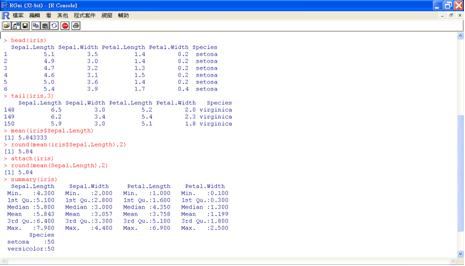### 二. 繪圖功能

plot函數可依參數的性質畫出不同的X-Y散佈圖、長條圖、盒狀圖、散佈圖矩陣：
>plot(Sepal.Length,Sepal.Width) 散佈圖
>plot(Species) 長條圖
>plot(Species,Sepal.Length) 盒狀圖
>plot(iris) 散佈圖矩陣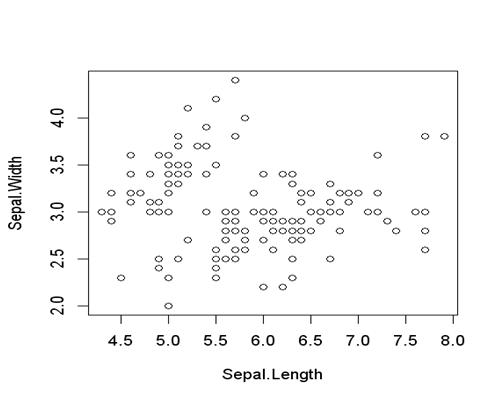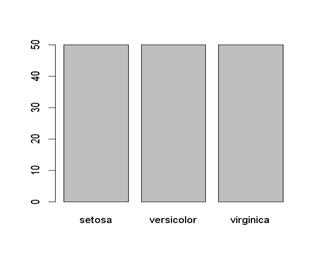>attach(iris)
>plot(Sepal.Length[Species=="setosa"],Petal.Length[Species=="setosa"], pch=1 ,col="black", xlim=c(4,8), ylim= c(0,8), main="classified scatter plot", xlab= "SLen", ylab= "PLen")
>points(Sepal.Length[Species=="virginica"], Petal.Length[Species== "virginica"],pch=3,col="green")
>points(Sepal.Length[Species=="versicolor"], Petal.Length[Species== "versicolor"],pch=2,col="red")
>legend(4,8,legend=c("setosa","versicolor","virginica"), col=c(1,2,3), pch=c(1,2,3))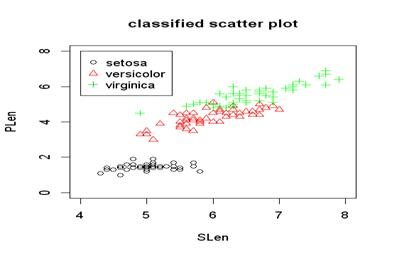### 三. 常態性檢定

attach(iris)
shapiro.test(Sepal.Length)
library(nortest)
qqnorm(Sepal.Length)
qqline(Sepal.Length,col="red")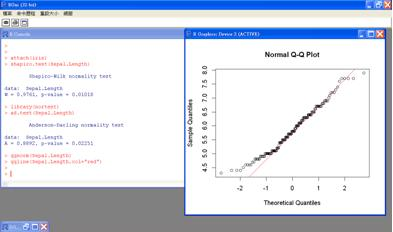hist(Sepal.Length,breaks=seq(4.0,8.0,0.25),prob=TRUE)### 四. 雙樣本均數檢定

xtabs(~Species)
setosa=subset(iris,Species=="setosa")
versicolor=subset(iris,Species=="versicolor")
var.test(setosa\$Petal.Width, versicolor\$Petal.Width)
t.test(setosa\$Petal.Width, versicolor\$Petal.Width, var.equal=FALSE)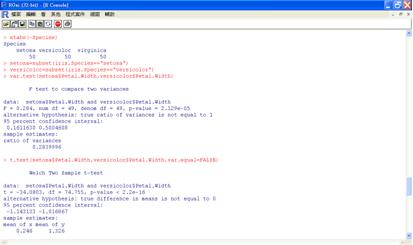iris2=subset(iris,Species!="virginica")
var.test(iris2\$Petal.Width ~ iris2\$Species)
t.test(iris2\$Petal.Width ~ iris2\$Species,var.equal=FALSE, conf=0.95)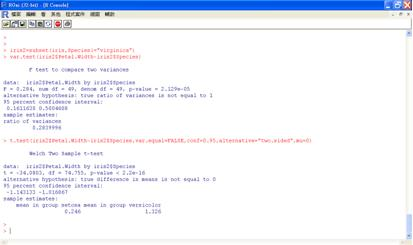### 五. 變異數分析

H0:μSetosa=μVersicolor=μVirginica
H1:至少有一種平均數和其他品種不相等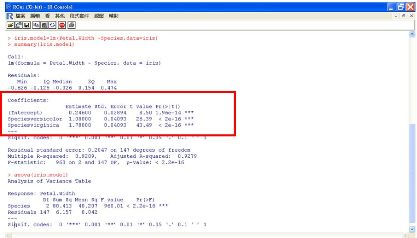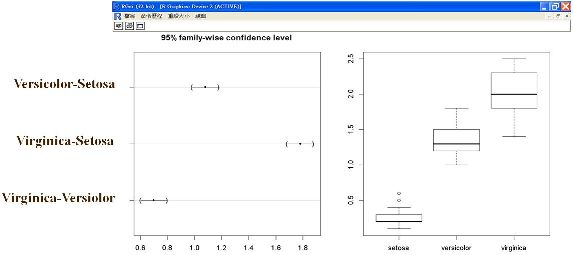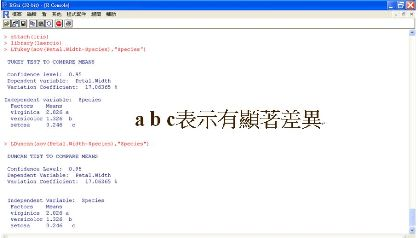### 六. 迴歸分析

model=lm(Y~X1+X2+…+Xk, data=…)Sepal.Length = 0.65084xSepal.Width + 0.70913xPetal.Length - 0.55648xPetal.Width + 1.85600

### 七. 決策樹資料探勘

n=0.1*nrow(iris)
test.index=sample(1:nrow(iris),n)
iris.train=iris[-test.index,]
iris.test=iris[test.index,]
library(tree)
iris.tree=tree(Species~ . ,data=iris.train)
iris.tree
plot(iris.tree)
text(iris.tree)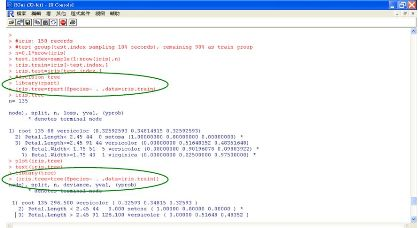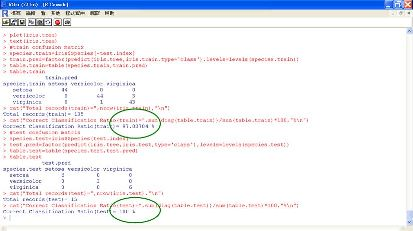#Random Forest
library(randomForest)
set.seed(777)
iris.rf=randomForest(Species ~ ., data=iris.train,importane=T,proximity=T)
print(iris.rf)
round(importance(iris.rf),2)
names(iris.rf)
(table.rf=iris.rf\$confusion)
sum(diag(table.rf)/sum(table.rf))
#predict
(rf.pred=predict(iris.rf,newdata=iris.test))
(table.test=table(Species=species.test,Predicted=rf.pred))
sum(diag(table.test)/sum(table.test))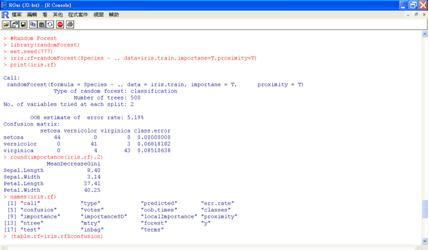(iris.urf=randomForest(iris[,-5]))
MDSplot(iris.urf,iris\$Species,palette=rep(1,3),pch=as.numeric(iris\$Species))